Drive de adds rolex uk new Cartier series, new moon phase rolex replica watches equipped with Cartire 1904-LU MC refining workshop movement, 6 position with fake omega moon phase display and the complex function of accurate reproduction of the fake hublot watches new moon, moon, moon and moon cycle replacement time.
World Championships @ Lift Up: Search Results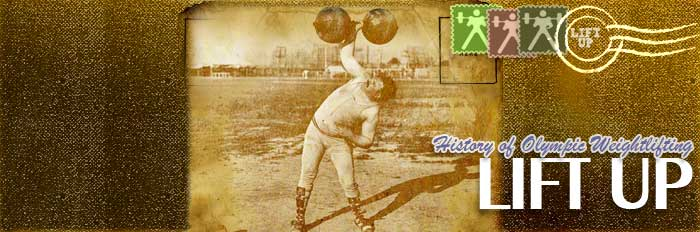## Lift Up: All-Time Best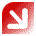The Lift Up project is brought to you by chidlovski.com.

D. Rigert, USSR

# World Championships from 1891 to Today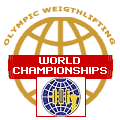Gold Medals at World Championships
Weight Class: SUPER HEAVYWEIGHT

Year  Class   Champion Results (kg)
1920 82.5+Filippo BOTTINO, Italy 70+75+120 = 265
1922 82.5+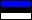Harald TAMMER, Estonia
1923 82.5+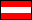Franz AIGNER, Austria 115+0+130 = 420
1924 82.5+Giuseppe TONANI, Italy 112.5+100+130 = 517.5
1928 82.5+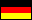Josef STRASSBERGER, Germany 122.5OR+107.5+142.5 = 372.5WR
1932 82.5+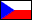Jaroslav SKOBLA, Czechoslovakia 112.5+115+152.5OR = 380OR
1936 82.5+Josef MANGER, Germany 132.5OR+122.5+155 = 410WR
1937 82.5+Josef MANGER, Germany 135+125+160 = 420
1938 82.5+Josef MANGER, Germany 135+122.5+152.5 = 410
1946 82.5+John DAVIS, United States 135+137.5+162.5 = 435WR
1947 82.5+John DAVIS, United States 140+140WR+175WR = 455WR
1948 82.5+John DAVIS, United States 137.5OR+137.5+177.5WR = 452.5OR
1949 82.5+John DAVIS, United States 137.5+140+165 = 442.5
1950 82.5+John DAVIS, United States 145+147.5+170 = 462.5WR
1951 90+John DAVIS, United States 142.4+130+160 = 432.5
1952 90+John DAVIS, United States 150OR+145OR+165 = 460OR
1953 90+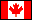Douglas HEPBURN, Canada 167.5+135+165 = 467.5
1954 90+Norbert SCHEMANSKY, United States 150+150+187.5 = 487.5
1955 90+Paul ANDERSON, United States 185+145+182.5 = 512.5
1956 90+Paul ANDERSON, United States 167.5+145EOR+187.5OR = 500OR
1957 90+Alexey MEDVEDEV, Soviet Union 165+147.5+187.5 = 500
1958 90+Alexey MEDVEDEV, Soviet Union 160+145+180 = 485
1959 90+Yury VLASOV, Soviet Union 160+147.5+192.5 = 500
1960 90+Yury VLASOV, Soviet Union 180OR+155OR+202.5WR = 537.5WR
1961 90+Yury VLASOV, Soviet Union 180+155+190 = 525
1962 90+Yury VLASOV, Soviet Union 177.5+155+207.5 = 540
1963 90+Yury VLASOV, Soviet Union 187.5+160+210 = 557.5
1964 90+Leonid ZHABOTINSKY, Soviet Union 187.5+167.5OR+217.5WR = 572.5OR
1965 90+Leonid ZHABOTINSKY, Soviet Union 185+165+202.5 = 552.5
1966 90+Leonid ZHABOTINSKY, Soviet Union 190+160+217.5 = 567.5
1967 90+Stanislav BATISHCHEV, Soviet Union 185+155+210 = 550
1968 90+Leonid ZHABOTINSKY, Soviet Union 200OR+170OR+202.5 = 572.5EOR
1969 110+Joseph DUBE, United States 202.5+162.5+212.5 = 577.5
1970 110+Vasily ALEXEEV, Soviet Union 215+170+227.5WR = 612.5
1971 110+Vasily ALEXEEV, Soviet Union 230WR+170+235WR = 635
1972 110+Vasily ALEXEEV, Soviet Union 235OR+175OR+230OR = 640OR
1973 110+Vasily ALEXEEV, Soviet Union 177.5+225 = 402.5
1974 110+Vasily ALEXEEV, Soviet Union 185+240 = 425
1975 110+Vasily ALEXEEV, Soviet Union 187.5+240 = 427.7
1976 110+Vasily ALEXEEV, Soviet Union 185OR+255WR = 440
1977 110+Vasily ALEXEEV, Soviet Union 185+245 = 430
1978 110+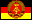Jurgen HEUSER, East Germany 185+232.5 = 417.5
1979 110+Sultan RAKHMANOV, Soviet Union 192.5+237.5 = 430
1980 110+Sultan RAKHMANOV, Soviet Union 195OR+245 = 440EOR
1981 110+Anatoly PISARENKO, Soviet Union 187.5+237.5 = 425
1982 110+Anatoly PISARENKO, Soviet Union 197.5+247.5 = 445
1983 110+Anatoly PISARENKO, Soviet Union 205+245 = 450
1984 110+Dean LUKIN, Australia 172.5+240 = 412.5
1985 110+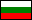Antonio KRASTEV, Bulgaria 202.5+235 = 437.5
1986 110+Antonio KRASTEV, Bulgaria 215+245 = 460
1987 110+Alexander KURLOVICH, Soviet Union 212.5+260 = 472.5
1988 110+Alexander KURLOVICH, Soviet Union 212.5OR+250 = 462.5OR
1989 110+Alexander KURLOVICH, Soviet Union 215+245 = 460
1990 110+Leonid TARANENKO, Soviet Union 195+255 = 450
1991 110+Alexander KURLOVICH, Soviet Union 205+250 = 455
1992 110+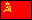Alexander KURLOVICH, Unified Team (CIS) 205+245 = 450
1993 108+Ronny WELLER, Germany 200+242.5 = 442.5
1994 108+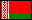Alexander KURLOVICH, Belarus 205+253 = 457.5
1995 108+Andrey CHEMERKIN, Russia 197.5+245 = 442.5
1996 108+Andrey CHEMERKIN, Russia 197.5+260 = 457.5
1997 108+Andrey CHEMERKIN, Russia 195+262.5 = 462.5
1998 105+Andrey CHEMERKIN, Russia 197.5+240 = 437.5
1999 105+Andrey CHEMERKIN, Russia 200+257.5 = 457.5
2000 105+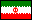Hossein REZAZADEH, Iran 212.5+260 = 472.5
2001 105+Saeed Salem JABER, Qatar 210+250 = 460
2002 105+Hossein REZAZADEH, Iran 210+263WR = 472.5
2003 105+Hossein REZAZADEH, Iran 207.5+250 = 457.5
2004 105+Hossein REZAZADEH, Iran 210+263.5WR = 472.5
2005 105+Hossein REZAZADEH, Iran 210+251 = 461
2006 105+Hossein REZAZADEH, Iran 202+246 = 448
2007 105+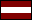Viktors SCHERBATIHS, Latvia 202+240 = 442
2008 105+Matthias STEINER, Germany 203+258 = 461
2009 105+Yong-Kwon AN, South Korea 198+247 = 445
2010 105+Behdad SALIMIKORDASIABI, Iran 208+245 = 453
2011 105+Behdad SALIMIKORDASIABI, Iran 214+250 = 464
2012 105+Behdad SALIMIKORDASIABI, Iran 208+247 = 455
2013 105+Ruslan ALBEGOV, Russia 209+255 = 464
2014 105+Ruslan ALBEGOV, Russia 210+252 = 462
2015 105+Lasha TALAKHADZE, Georgia 207+247 = 454
2016 105+Lasha TALAKHADZE, Georgia 215+258 = 473
2017 105+Lasha TALAKHADZE, Georgia 220+257 = 477
2018 109+Lasha TALAKHADZE, Georgia 217WR+257WR = 474WR
2019 109+Lasha TALAKHADZE, Georgia 220WR+264WR = 484WR
2020 109+Lasha TALAKHADZE, Georgia 223WR/0R+265WR/0R = 488WR/0R
2021 109+Lasha TALAKHADZE, Georgia 225WR+267WR = 492WR

Note: To view a complete list of the winners of a specific world championship above,